# MATLAB Modelling of Heat Equation

April 29, 2022

By  Shubham

lang: en_US

## Introduction:

According to Wikipedia, a partial differential equation (PDE) is an equation that imposes relations between the various partial derivatives of a multivariable function. PDE forms the foundational basis for modern scientific understanding of sound, heat, diffusion, thermodynamics, and quantum mechanics. This blog will deal with applying partial differential equations in the form of the heat equation and its modelling in MATLAB.

## The Heat Equation: Mathematical Preliminaries

### Obtaining the heat equation:

Let’s start with the derivation of the heat equation. Consider a heat-conducting homogeneous rod, extending from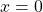to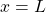along theaxis having a uniform cross-section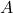.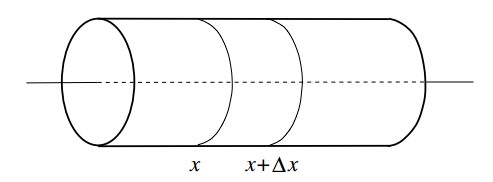Heat conduction in a thin bar

Let the material density be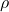. Assume the rod is laterally insulated so that heat flows only in the direction of theaxis. Let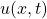denote the temperature of the cross-section at point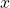at any instant of time t and sigma be the specific heat of the rod.

The amount of heat in the rod between the cross-section atand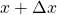is,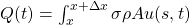Rate of heat flowing into the segmentis,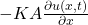Rate of heat flowing out of the segmentis,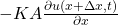Heredenotes the thermal conductivity of the rod. You might be wondering about the negative sign in front of the two equations given above. The negative sign indicates that the heat flows in the direction of colder regions. The amount of heat content between the sectionandmust be equal to the difference between the amount of heat that flows in at cross-sectionand the amount heat that flows out the at cross-section. Hence,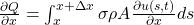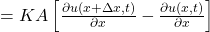To solve the integration, assume the function to be a continuous function of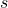and apply the mean value theorem.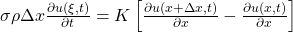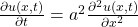This equation is called one-dimensional heat equation. Here,. This constant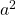is called the diffusivity within the solid.

### Initial and boundary conditions:

An initial boundary value problem for the heat equation consists of the PDE along with three other conditions specified at,and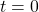. More formally it can be written as: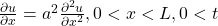and it describes the temperature distribution in the rod at any time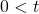subject to conditionsAnd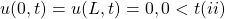Equation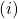describes the initial conditions while equationdescribes the boundary conditions.

### Solving the heat equation:

let’s reconsider our heat equation. We have to find the solution to the homogeneous heat equation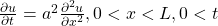Having the initial condition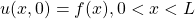and boundary conditions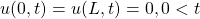.

We will use the variable separation method to solve the equation. The solution that we seek will be in the form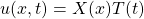Since,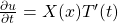and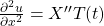. Therefore, the above equation becomes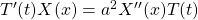. This equation can be rewritten as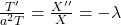. Here,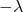is a constant. This equation gives us two ordinary differential equations: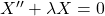and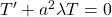. The boundary condition changes toand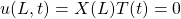for.

Depending on the value of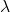, there are three cases: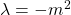,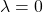and. For all the three cases the general solution for the first differential equation will be as follows:

• For,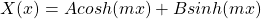• For,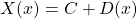• For,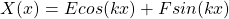For second differential equation, we will use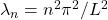in the equation. The general solution is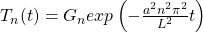.

The particular solution of the considered heat equation is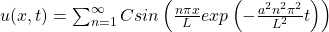. The general solutions hence equal a linear sum of the particular solution. Here the coefficient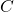can be obtained by settingfor the entire equation. Hence,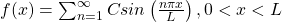And,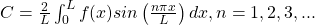Now that the heat equation is ready let’s model it in MATLAB.

The initialization conditions and boundary values are based on the heat equation we used and derived earlier. Hence, if the boundary conditions are different, they should be changed in the codes. With this info in mind, let’s dive into the MATLAB code.

## Modelling the heat equation in MATLAB:

%%%%%%%%%%%%%%%%%%%%%%%%%%%%%%%%%%%%%%%%%%%%%
% Author: Shubham Kumar, MATLAB Helper
% Topic: Heat Equation
% Website: https://matlabhelper.com
% Date: 03-04-2022
% Version: R2021b
%%%%%%%%%%%%%%%%%%%%%%%%%%%%%%%%%%%%%%%%%%%%%

clc;
clear;
close all;

% Initialisation
M = 15;
dx = 0.125;
dt = 0.05;
X = 0:dx:pi;
T = 0:dt:2;
u = zeros(length(T),length(X));

% Boundary Conditions
Xx = repmat(X,[length(T) 1]);
Tt = repmat(T',[1 length(X)]);

% Solving the equations
for m = 1:M
temp = 2*m-1;
coeff = 8 / (pi * temp * temp * temp);
u = u + coeff * sin(temp*Xx) .* exp(-temp * temp * Tt);
end

% Plotting the heat equation
surf(Xx,Tt,u)
xlabel('distance','Fontsize',20);
ylabel('time','Fontsize',20);
zlabel('u(x,t)','Fontsize',20);

Seeing the code above, one might wonder what the repmat() function is and why it is used. As per the MathWorks website, the repmet() function returns copies of an array. Hence, we are making copies of thefunction for the different values of X and T. To solve the equations, we will introduce a for loop which will go up to the value of M and calculates the coefficients and hence the function.

Finally, let’s plot the equations and check our result.

On running the above code, MATLAB will generate the following graph: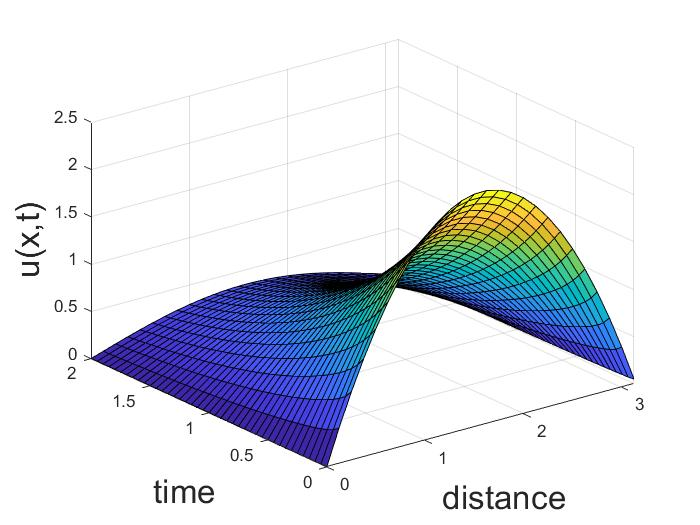Graph of heat equation

## Analyzing the result:

Since the result is a 3D plot, it can be rotated to a different point of view and analyzed. The unrotated plot tells us that temperaturewithin a thin bar is zero at the ends. This result satisfies the boundary conditions. Now, let’s rotate the graph.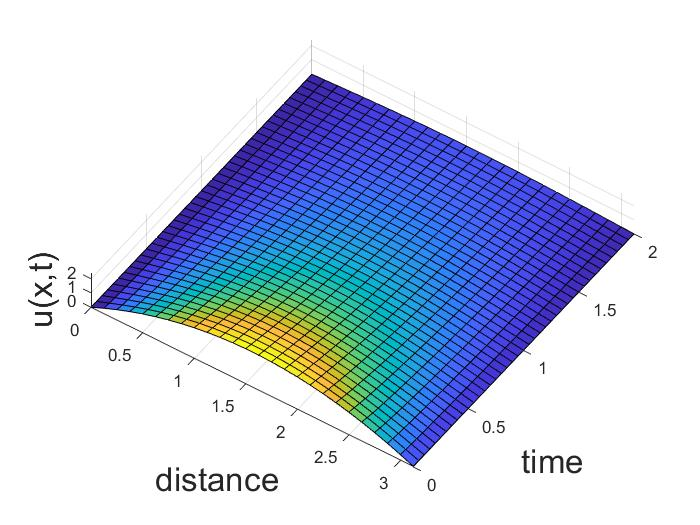Graph of heat equation rotated horizontally

The graph shows that the heat flows out from the center of the thin metallic bar towards the colder region and the ends of the metallic bar where the temperature is removed.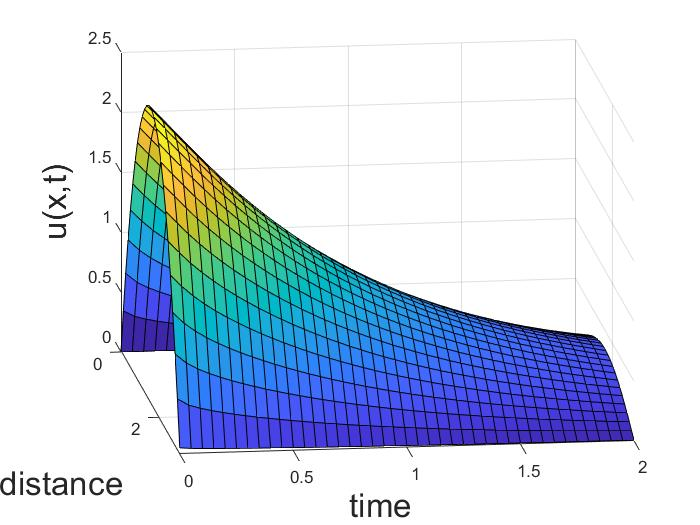Graph of heat equation rotated sidewise

The parabolic shape is reduced as we move away from the hotter region towards the colder area with the increase in time. This again shows that the heat is being removed from the ends of the metallic bar.

Ready to take your MATLAB skills to the next level? Look no further! At MATLAB Helper, we've got you covered. From free community support to expert help and training, we've got all the resources you need to become a pro in no time. If you have any questions or queries, don't hesitate to reach out to us. Simply post a comment below or send us an email at [email protected].

And don't forget to connect with us on LinkedIn, Facebook, and Subscribe to our YouTube Channel! We're always sharing helpful tips and updates, so you can stay up-to-date on everything related to MATLAB. Plus, if you spot any bugs or errors on our website, just let us know and we'll make sure to fix it ASAP.

Ready to get started? Book your expert help with Research Assistance plan today and get personalized assistance tailored to your needs. Or, if you're looking for more comprehensive training, join one of our training modules and get hands-on experience with the latest techniques and technologies. The choice is yours – start learning and growing with MATLAB Helper today!

Education is our future. MATLAB is our feature. Happy MATLABing!

#### Shubham

I have done my bachelor degree in electronics from University of Delhi. I like to tinker with the hardware to understand how it actually works.

#### MATLAB Helper ®

Use Website Chat or WhatsApp at +91-8104622179